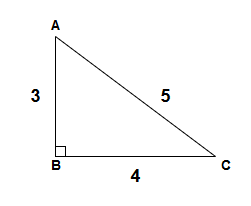﻿ ABC116 A - Right Triangle - Atcoder

# Home

Score : $100$ points

### Problem Statement

There is a right triangle $ABC$ with $∠ABC=90°$.

Given the lengths of the three sides, $|AB|,|BC|$ and $|CA|$, find the area of the right triangle $ABC$.

It is guaranteed that the area of the triangle $ABC$ is an integer.

### Constraints

• $1 \leq |AB|,|BC|,|CA| \leq 100$
• All values in input are integers.
• The area of the triangle $ABC$ is an integer.

### Input

Input is given from Standard Input in the following format:

$|AB|$ $|BC|$ $|CA|$


### Output

Print the area of the triangle $ABC$.

### Sample Input 1

3 4 5


### Sample Output 1

6This triangle has an area of $6$.

### Sample Input 2

5 12 13


### Sample Output 2

30


This triangle has an area of $30$.

### Sample Input 3

45 28 53


### Sample Output 3

630


This triangle has an area of $630$.